# New Tribonacci recurrence relations and addition formulas

Notes on Number Theory and Discrete Mathematics
Print ISSN 1310-5132, Online ISSN 2367-8275
Volume 26, 2020, Number 4, Pages 164—172
DOI: 10.7546/nntdm.2020.26.4.164-172

## Details

### Authors and affiliations

Kunle AdegokeDepartment of Physics and Engineering Physics, Obafemi Awolowo University
220005 Ile-Ife, Nigeria

Adenike OlatinwoDepartment of Physics and Engineering Physics, Obafemi Awolowo University
220005 Ile-Ife, Nigeria

Winning OyekanmiDepartment of Physics and Engineering Physics, Obafemi Awolowo University
220005 Ile-Ife, Nigeria

### Abstract

Only one three-term recurrence relation, namely,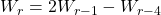, is known for the generalized Tribonacci numbers,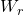,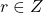, defined byand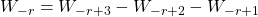, where,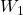and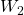are given, arbitrary integers, not all zero. Also, only one four-term addition formula is known for these numbers, which is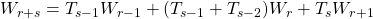, whereis the Tribonacci sequence, a special case of the generalized Tribonacci sequence, with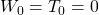and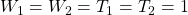. In this paper we discover three new three-term recurrence relations and two identities from which a plethora of new addition formulas for the generalized Tribonacci numbers may be discovered. We obtain a simple relation connecting the Tribonacci numbers and the Tribonacci–Lucas numbers. Finally, we derive quadratic and cubic recurrence relations for the generalized Tribonacci numbers.

### Keywords

• Tribonacci number
• Tribonacci–Lucas number
• Recurrence relation

• 11B39
• 11B37

### References

1. Adegoke, K. (2018). Weighted Tribonacci sums, arXiv:1804.06449[math.CA].
2. Atanassova, L. (2019). A remark on the Tribonacci sequences, Notes on Number Theory and Discrete Mathematics, 25(3), 138–141.
3. Feng, J. (2011). More identities on the Tribonacci numbers, Ars Combinatoria, 100, 73–78.
4. Frontczak, R. (2018). Convolutions for generalized Tribonacci numbers and related results, International Journal of Mathematical Analysis, 12 (7), 307–324.
5. Frontczak, R. (2018). Sums of Tribonacci and Tribonacci–Lucas numbers, International Journal of Mathematical Analysis, 12(1), 19–24.
6. Gabai, H. (1970). Generalized Fibonacci k-sequences, The Fibonacci Quarterly, 8(1), 31–38.
7. Komatsu, T. (2011). On the sum of reciprocal Tribonacci numbers, Ars Combinatoria, 98, 447–459.
8. Shah, D. V. (2011). Some Tribonacci identities, Mathematics Today, 27, 1–9.
9. Waddill, M. E., & Sacks, L. (1967). Another generalized Fibonacci sequence, The Fibonacci Quarterly, 5(3), 209–222.

## Cite this paper

Adegoke, K., Olatinwo, A., & Oyekanmi, W. (2020). New Tribonacci recurrence relations and addition formulas. Notes on Number Theory and Discrete Mathematics, 26 (4), 164-172, doi: 10.7546/nntdm.2020.26.4.164-172.